Ch 1. Limits Multimedia Engineering Math Limit of aSequence Limit of aFunction LimitLaws Continuity RateChange
 Chapter 1. Limits 2. Derivatives I 3. Derivatives II 4. Mean Value 5. Curve Sketching 6. Integrals 7. Inverse Functions 8. Integration Tech. 9. Integrate App. 10. Parametric Eqs. 11. Polar Coord. 12. Series Appendix Basic Math Units Search eBooks Dynamics Fluids Math Mechanics Statics Thermodynamics Author(s): Hengzhong Wen Chean Chin Ngo Meirong Huang Kurt Gramoll ©Kurt GramollMATHEMATICS - THEORY Function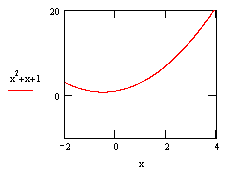The quantity relationship of function y = x2 + x + 1 If the quantity of y is determined by another value x, then y is a function of x. If f denotes the function, then the formula y = f(x) indicate y depends on x. Thus y is a dependent variable and x is a independent variable. An example of a function would be:      y = x2 + x + 1 The diagram on the left shows that the quantity of y which is equal to x2 + x + 1 depends on the value of x. Limit of a Function If for each positive ε, no matter how small, there is a corresponding positive δ such that if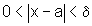thenIn this situation, the limit of f(x) is A as x approaches a, and it can be written as: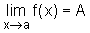Limits Involving Infinity In this segment, three kind of situations will be discussed.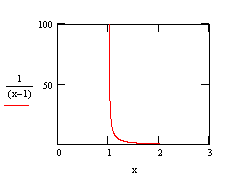The limit of f(x) is infinity when x approaches 1 The limit of f(x) is ∞ as x approaches a if for each number B, no matter how large, there is a corresponding positive δ, such that ifthen      f(x) > B Its notation is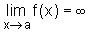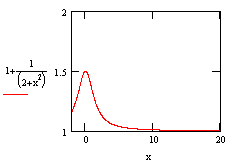The limit of f(x) is 1 when x approaches +∞ The limit of f(x) is A as x approaches ∞ if for each positive ε, no matter how small, there is a corresponding B such that if        x > B thenIts notation is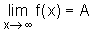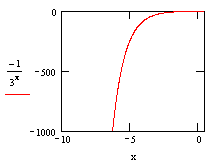The limit of f(x) is -∞ when x approaches -∞ The limit of f(x) is -∞ as x approaches -∞ if for each number B, no matter how large, there is a corresponding C, such that if        x< -C then      f(x) < -B Its notation is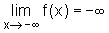Summary Summary This section introduces limit of a function and three cases involving infinity. When discussing limit of a function, it must be the limit of a specific point.